# Stratigraphic diagrams

#### 2023-01-17

This vignette covers creating stratigraphic diagrams using ggplot2, highlighting the helpers contained within tidypaleo. The ggplot2 framework was chosen because it is quite flexible and can be used to create almost any time-stratigraphic diagram except ones that involve multiple axes (we can have a fight about whether or not those are appropriate anyway, but if you absolutely need to create them I suggest you look elsewhere). It will help considerably if you are already familiar with ggplot2 (a great tutorial can be found here).

First, you will need to load the packages. For this tutorial, I will use tidypaleo, and the tidyverse (this includes ggplot2). Later, I will use patchwork to create more complex plots. I prefer the `theme_paleo()` theme for stratigraphic diagrams using ggplot2, so I will set this here as well. Setting the base font size to a small value (8 pt in this case) is useful for our purposes.

``````library(tidyverse)
library(tidypaleo)
theme_set(theme_paleo(8))``````

Next, you will need to load the example data. This vignette uses 4 datasets that are already in the correct format for plotting. These are datasets in the tidypaleo package: `long_lake_plottable`, `alta_lake_geochem`, `keji_lakes_plottable`, and `halifax_lakes_plottable`. These represent four common dataset types for which stratigraphic plots are created.

``````data("long_lake_plottable")
data("alta_lake_geochem")
data("keji_lakes_plottable")
data("halifax_lakes_plottable")``````

## Geochemical stratigraphic plots

Geochemical stratigraphic plots include any kind of plot where each panel of the plot should be scaled to the minimum and maximum of the data. This includes (most) non-species abundance data. The example data, `alta_lake_geochem`, is a data frame in parameter-long (one row per measurement) form (you may need to use `gather()` to get your data into this form, which you can learn about in this tutorial). These measurements are from Alta Lake, British Columbia (Canada), and you can read more about them in the journal article.

``````alta_lake_geochem
#> # A tibble: 192 × 9
#>    location param depth   age value stdev units     n zone
#>    <chr>    <chr> <dbl> <dbl> <dbl> <dbl> <chr> <int> <chr>
#>  1 ALGC2    Cu     0.25 2015.  76   NA    ppm       1 Zone 3
#>  2 ALGC2    Cu     0.75 2011. 108.   4.50 ppm       3 Zone 3
#>  3 ALGC2    Cu     1.25 2008. 158   NA    ppm       1 Zone 3
#>  4 ALGC2    Cu     1.75 2003. 169   NA    ppm       1 Zone 3
#>  5 ALGC2    Cu     2.5  1998. 161   NA    ppm       1 Zone 3
#>  6 ALGC2    Cu     3.5  1982. 129   NA    ppm       1 Zone 3
#>  7 ALGC2    Cu     4.5  1966.  88.7  3.86 ppm       3 Zone 2
#>  8 ALGC2    Cu     5.5  1947.  65   NA    ppm       1 Zone 2
#>  9 ALGC2    Cu     6.5  1922.  62.3  9.53 ppm       3 Zone 2
#> 10 ALGC2    Cu     7.5  1896.  48   NA    ppm       1 Zone 2
#> # … with 182 more rows``````

Plotting a single core with `ggplot()` involves several steps:

• The initial `ggplot()` call, where we set which columns will get mapped to the `x` and `y` values for each layer.
• Two layers: `geom_lineh()` and `geom_point()`. We use `geom_lineh()` because our plots will be oriented vertically (`geom_line()` is designed for horizontal plots).
• Specify how data are divided between facets using `facet_geochem_gridh()` (we use `_gridh()` to orient the panels horizontally rather than vertically).
• Reverse the Y-axis, so that a depth of 0 is at the top.
• Remove the X label, and set the Y label to “Depth (cm)”.
``````alta_plot <- ggplot(alta_lake_geochem, aes(x = value, y = depth)) +
geom_lineh() +
geom_point() +
scale_y_reverse() +
facet_geochem_gridh(vars(param)) +
labs(x = NULL, y = "Depth (cm)")

alta_plot``````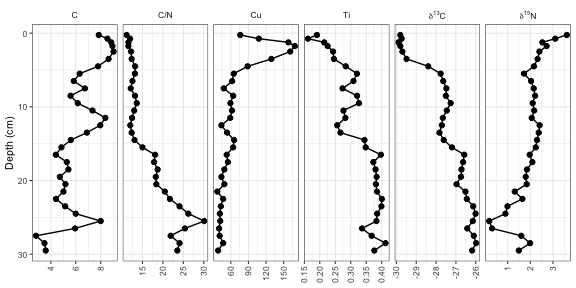That’s it! Of course, there are many modifications that can be made, which are covered in the next few sections.

It is common to highlight certain depths or ages on strat diagrams, or to add things to various places on a plot. This is accomplished by adding additional layers to the plot. For example, highlighting the depth at which the zones change (according to the paper, this is at 4 cm and 16 cm).

``````alta_plot +
geom_hline(yintercept = c(4, 16), col = "red", lty = 2, alpha = 0.7)``````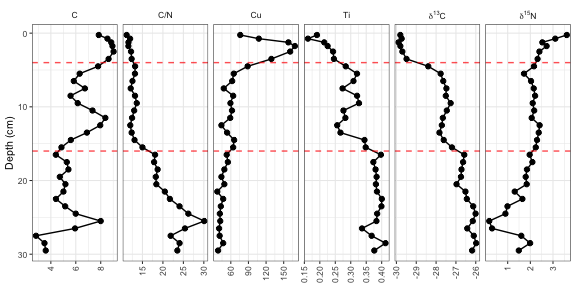The `geom_hline()` function creates its own data using the `yintercept` argument, but other geometries require us to create our own data. For example, highlighting the middle zone using `geom_rect()` would look like this:

``````zone_data <- tibble(ymin = 4, ymax = 16, xmin = -Inf, xmax = Inf)
alta_plot +
geom_rect(
mapping = aes(ymin = ymin, ymax = ymax, xmin = xmin, xmax = xmax),
data = zone_data,
alpha = 0.2,
fill = "blue",
inherit.aes = FALSE
)``````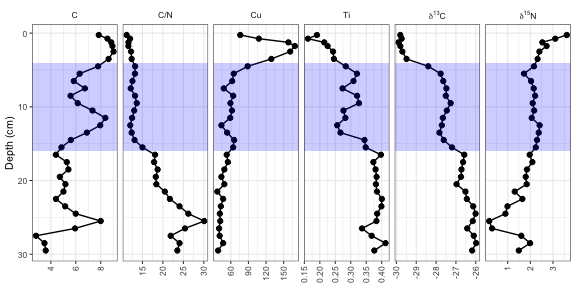So far, each new layer we have added has been propogated to all facets. To restrict annotations to a specific facet, we need to include the facetting column (in this case, `param`) in the layer data. The element copper (Cu) has standards set by the Canadian government (ISQG of 35.7 ppm), and highlighting values that are outside that range can be done in a similar way to highlighting the middle zone.

``````cu_standard_data <- tibble(param = "Cu", xmin = 35.7, xmax = Inf, ymin = -Inf, ymax = Inf)
alta_plot +
geom_rect(
mapping = aes(ymin = ymin, ymax = ymax, xmin = xmin, xmax = xmax),
data = cu_standard_data,
alpha = 0.2,
fill = "red",
inherit.aes = FALSE
)``````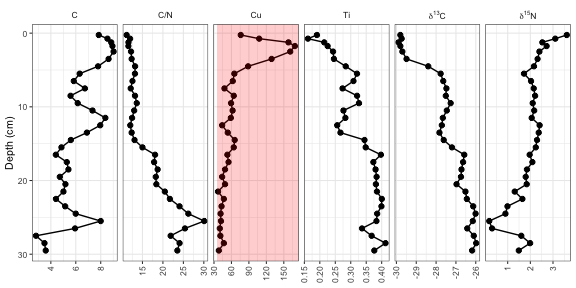To highlight the syntax that changed from the original plot, I’ve been using `alta_plot + ...`, however with layers such as these, it may be desirable to have the annotation appear under the original data. To do this, you would have to add your annotation layer (`geom_rect()`) before your data layer (`geom_lineh()` and `geom_point()`).

### Error bars

In the `alta_lake_geochem` data frame, there is a column for standard deviation (`stdev`). You can plot these values as error bars using `geom_errorbarh()`.

``````alta_plot +
geom_errorbarh(aes(xmin = value - stdev, xmax = value + stdev), height = 0.5)``````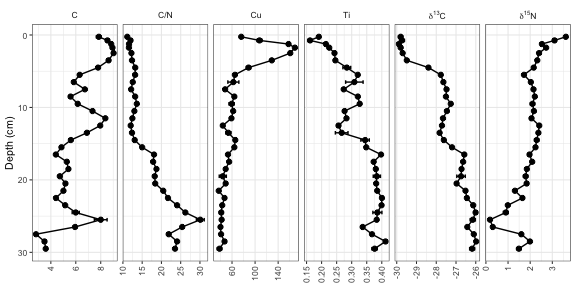### Reordering facets

To control the order of the facets, the column on which they are facetted (`param`) must be a `factor()`, where the `levels` indicate the order. I suggest using `fct_relevel()` to do this, so that you only need to specify which items should go first. Usually I do this using a pipe (`%>%`) and `mutate()` to avoid assigning any intermediate variables.

``````alta_lake_geochem %>%
mutate(param = fct_relevel(param, "Ti", "Cu", "C/N")) %>%
ggplot(aes(x = value, y = depth)) +
...``````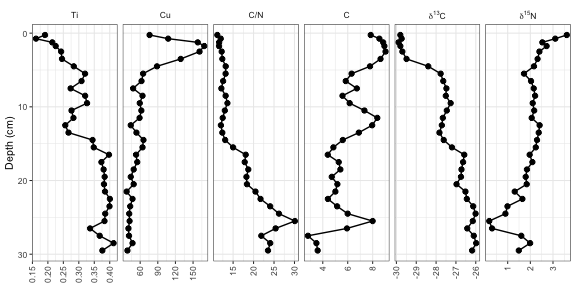### Plotting a subset of the data

The `halifax_geochem` data frame was designed so that it is a reasonable number of parameters to plot at one time. Usually you will have a data frame with many more parameters, which you can subset using `filter()` before calling `ggplot()`. Usually I do this using a pipe (`%>%`) to avoid assigning any intermediate variables.

``````alta_lake_geochem %>%
filter(param %in% c("d15N", "d13C", "C/N")) %>%
ggplot(aes(x = value, y = depth)) +
...``````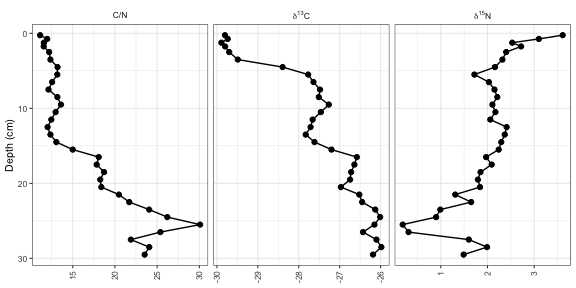### Using a y-axis with ages and depths

When plotting a single core, it is good practice to plot both ages and depths in the core. In ggplot2, this comes as a second axis generated by a helper function, `scale_y_depth_age()`, that uses an `age_depth_model()` to produce a second axis. For Alta Lake, the age depth model as published is included in the `alta_lake_bacon_ages` object. It’s possible to set the age breaks using the `age_breaks` argument, and the labels using the `age_labels` argument (this is useful for adding error information to ages on a second axis). The axis title can be set using the `age_name` argument.

``````alta_adm <- age_depth_model(
alta_lake_bacon_ages,
depth = depth_cm,
age = 1950 - age_weighted_mean_year_BP
)

alta_plot +
scale_y_depth_age(
)``````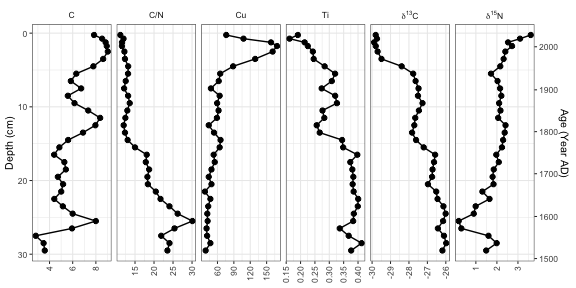### Adding units to facet labels

Ideally, we’d like to specify the unit in which each of these parameters was measured. This can be done (1) by renaming each parameter before it gets to `ggplot()`, or (2) by using the `units` argument of the `facet_geochem_*()` functions. I suggest using the second option because it maintains the order of the parameters that you’ve chosen, and keeps the automatic reformatting of `d13C`. The `units` argument takes a named character vector, which you can create using `c("parameter" = "unit")`. If most paremeters are in the same unit, you can pass the default unit using `default_unit = "unit"`, and if you need to suppress a unit for an item, you can use `NA`.

``````alta_plot +
facet_geochem_gridh(
vars(param),
units = c("C/N" = NA, "Cu" = "ppm", "d13C" = "‰", "d15N" = "‰"),
default_units = "%"
)``````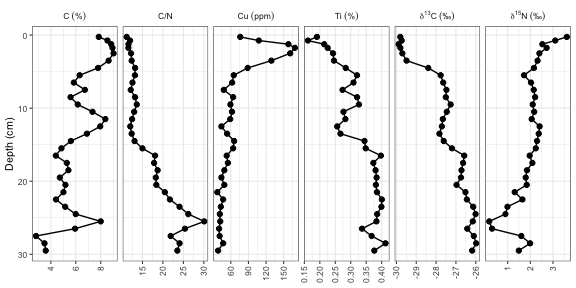### Multiple cores

So far we’ve plotted one core. That’s great, but often there is more than one core that needs to be plotted. There are two ways to go about this depending on how you would like identical parameters for each lake to be compared: using `facet_geochem_gridh()` will stretch the axis limits to fit values from both cores, and creating (and combining) two separate plots using `patchwork::wrap_plots()` will result in each panel stretching to the min/max of the data for that parameter. We’ll illustrate both of these using some other data from Long Lake, Nova Scotia.

``````combined_data <- bind_rows(long_lake_plottable, alta_lake_geochem)
combined_data
#> # A tibble: 243 × 12
#>    dataset     location param depth value     sd units zone      n n_det…¹   age
#>    <chr>       <chr>    <chr> <dbl> <dbl>  <dbl> <chr> <chr> <int>   <int> <dbl>
#>  1 long_lake17 LL PC2   C/N       1  15.4 NA     <NA>  Unit…     1       1    NA
#>  2 long_lake17 LL PC2   C/N       6  15.8  0.290 <NA>  Unit…     2       2    NA
#>  3 long_lake17 LL PC2   C/N      11  24.0 NA     <NA>  Unit…     1       1    NA
#>  4 long_lake17 LL PC2   C/N      16  21.4 NA     <NA>  Unit…     1       1    NA
#>  5 long_lake17 LL PC2   C/N      21  23.2 NA     <NA>  Unit…     1       1    NA
#>  6 long_lake17 LL PC2   C/N      26  25.4 NA     <NA>  Unit…     1       1    NA
#>  7 long_lake17 LL PC2   C/N      43  27.2 NA     <NA>  Unit…     1       1    NA
#>  8 long_lake17 LL PC2   C/N      48  24.3 NA     <NA>  Unit…     1       1    NA
#>  9 long_lake17 LL PC2   C/N      53  30.2 NA     <NA>  Unit…     1       1    NA
#> 10 long_lake17 LL PC2   C/N      63  24.4 NA     <NA>  Unit…     1       1    NA
#> # … with 233 more rows, 1 more variable: stdev <dbl>, and abbreviated variable
#> #   name ¹​n_detect``````

First we’ll go over the `facet_geochem_gridh()` approach, which adds `location` as another grouping variable. This will align parameters with themselves vertically, with one row on the plot per core. This usually the best option, as it makes values from one plot to another be directly comparable.

``````ggplot(combined_data, aes(x = value, y = depth)) +
geom_lineh() +
geom_point() +
scale_y_reverse() +
facet_geochem_gridh(vars(param), grouping = vars(location), scales = "free") +
labs(x = NULL, y = "Depth (cm)")``````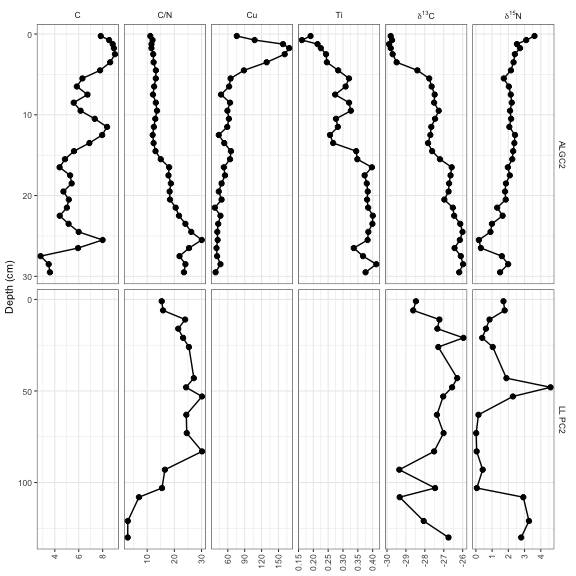The other way to do this is to create two separate plots, and combine them using the patchwork package. This is a good option when values from one core to another are so different that placing them on the same axis results in trends being obscured, or when ages and depths are absolutely necessary for both plots (using `scale_y_depth_age()` doesn’t work when combining locations using a facet).

``````alta_plot_1 <- combined_data %>%
filter(location == "ALGC2") %>%
ggplot(aes(x = value, y = depth)) +
geom_lineh() +
geom_point() +
scale_y_reverse() +
facet_geochem_gridh(vars(param), scales = "free") +
labs(x = NULL, y = "Depth (cm)", title = "Alta Lake")

long_plot_2 <- combined_data %>%
filter(location == "LL PC2") %>%
ggplot(aes(x = value, y = depth)) +
geom_lineh() +
geom_point() +
scale_y_reverse() +
facet_geochem_gridh(vars(param), scales = "free") +
labs(x = NULL, y = "Depth (cm)", title = "Long Lake")

library(patchwork)
wrap_plots(alta_plot_1, long_plot_2, ncol = 1)``````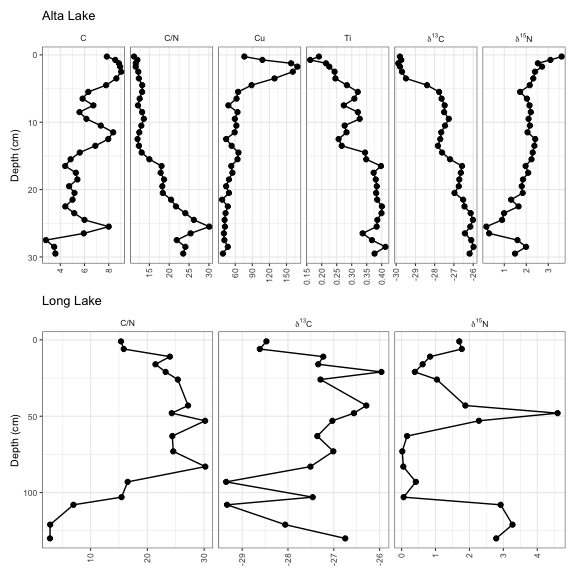It’s fairly common to see a CONISS dendrogram at the right of plots, and so tidypaleo wouldn’t be complete if it couldn’t make that happen. For a variety of technical reasons, you have to specify that you need `depth` mapped to the `y` aesthetic for both `layer_zone_boundaries()` and `layer_dendrogram()`. Note that you’ll have to do CONISS using `nested_chclust_coniss()`, which uses the rioja package to do the constrained cluster analysis. By default, this will calculate the number of plausible zones based on a broken stick simulation.

``````coniss <- alta_lake_geochem %>%
nested_data(qualifiers = c(age, depth), key = param, value = value, trans = scale) %>%
nested_chclust_coniss()

alta_plot +
layer_dendrogram(coniss, aes(y = depth), param = "CONISS") +
layer_zone_boundaries(coniss, aes(y = depth))``````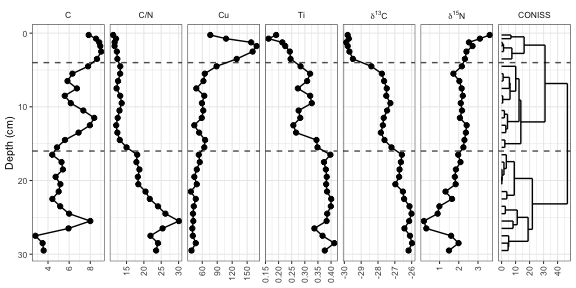### Using `facet_geochem_wraph()`

If you are familiar with `facet_grid()` in ggplot2, you will notice that `facet_geochem_gridh()` has done a few things differently, including automatically renaming `d13C` and `d15N` to the proper formatting. This function also rotates axis labels, because this is the only sure-fire way to make sure they don’t overlap. You can un-rotate them using `rotate_axis_labels = 0`, and you can wrap panels in a defined number of rows or columns using `facet_geochem_wraph()`. This works well for plotting a larger number of parameters when there is only one core involved.

``````alta_plot +
facet_geochem_wraph(vars(param), rotate_axis_labels = 0, ncol = 4)``````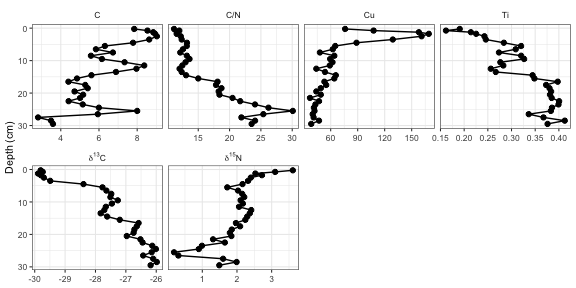### Going horizontal

Most stratigraphic diagrams are vertical, which is a reflection on the orientation of the core from which the data came. In many cases (especially when age is one of the axes), it may be adventageous to have the plots be horizontal, with time running from left to right along the x-axis. A few things need to change from our original plot, including the mapping, the line geometry, and the facet specification. Here I’ve used `facet_geochem_grid()` to stack the plots vertically, but you could also use `facet_geochem_wrap()`.

``````ggplot(alta_lake_geochem, aes(x = age, y = value)) +
geom_line() +
geom_point() +
scale_y_reverse() +
facet_geochem_grid(vars(param)) +
labs(x = "Age (Year AD)", y = NULL)``````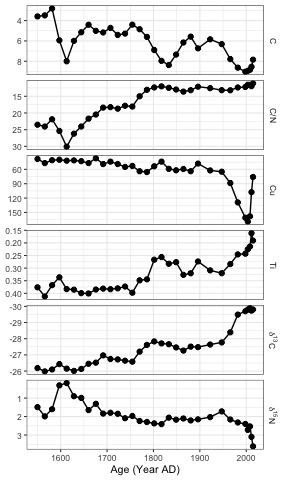## Species abundance stratigraphic plots

If our data is relative species abundance, there are a few more restrictions on the plot: each facet must represent values such that 10% abundance on one facet is equal to 10% in another facet, and facet names are often long enough that they must be rotated. The sample data for these exercises is `keji_lakes_plottable`, which again is in the form of one row per measurement. This dataset contains two lakes, Beaverskin Lake and Peskawa Lake, both located within Keji National Park, Nova Scotia, Canada (Ginn et al. 2007).

``````data("keji_lakes_plottable")
keji_lakes_plottable
#> # A tibble: 202 × 5
#>    location        depth taxon                                     count rel_a…¹
#>    <chr>           <dbl> <fct>                                     <dbl>   <dbl>
#>  1 Beaverskin Lake 0.125 Asterionella ralfsii var. americana (lar…     0    0
#>  2 Beaverskin Lake 0.125 Aulacoseira distans                           7    3.02
#>  3 Beaverskin Lake 0.125 Aulacoseira lirata                            4    1.72
#>  4 Beaverskin Lake 0.125 Cyclotella stelligera                         8    3.45
#>  5 Beaverskin Lake 0.125 Tabellaria flocculosa (strain III)            0    0
#>  6 Beaverskin Lake 0.125 Other                                       213   91.8
#>  7 Beaverskin Lake 0.375 Asterionella ralfsii var. americana (lar…     0    0
#>  8 Beaverskin Lake 0.375 Aulacoseira distans                           8    3.25
#>  9 Beaverskin Lake 0.375 Aulacoseira lirata                            5    2.03
#> 10 Beaverskin Lake 0.375 Cyclotella stelligera                        14    5.69
#> # … with 192 more rows, and abbreviated variable name ¹​rel_abund``````

The code to generate the plot is similar to our previous plots, except we use `geom_col_segsh()` (which instead of points or a path, draws lines connected to the x-axis), and `facet_abundanceh()` (which takes care of rotating facet labels, partially italicizing them, and setting the scales for each panel to start at 0.

``````keji_plot <- ggplot(keji_lakes_plottable, aes(x = rel_abund, y = depth)) +
geom_col_segsh() +
scale_y_reverse() +
facet_abundanceh(vars(taxon), grouping = vars(location)) +
labs(x = "Relative abundance (%)", y = "Depth (cm)")

keji_plot
#> Warning: Using the `size` aesthetic in this geom was deprecated in ggplot2 3.4.0.
#> ℹ Please use `linewidth` in the `default_aes` field and elsewhere instead.``````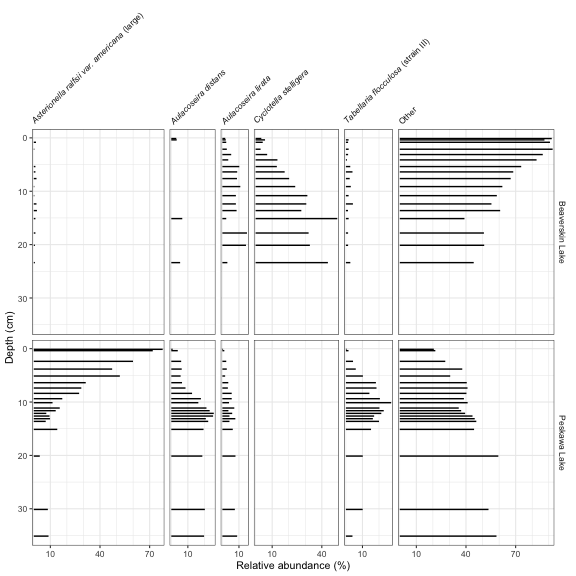Most of the modifications you can make on these plots are identical to the modifications you can make on non-abundance plots (see previous section), except for a few that we describe below.

### Different styles

There are a few famous styles for abundance plots, because a few existing programs (like Tilia, C2, and rioja) have some very specific defaults that show up on repeat in the literature. These styles can be replicated by changing or adding to `geom_col_segsh()`. For example, the classic Tilia “area” style for pollen diagrams:

``````ggplot(keji_lakes_plottable, aes(x = rel_abund, y = depth)) +
geom_areah() +
scale_y_reverse() +
facet_abundanceh(vars(taxon), grouping = vars(location)) +
labs(x = "Relative abundance (%)", y = "Depth (cm)")``````